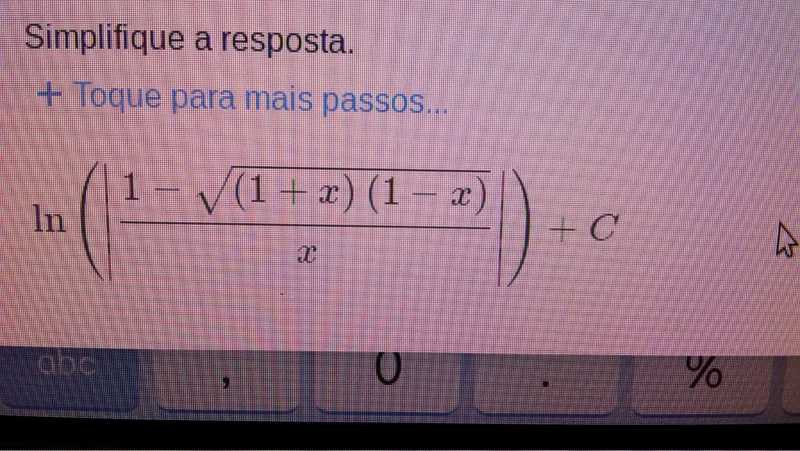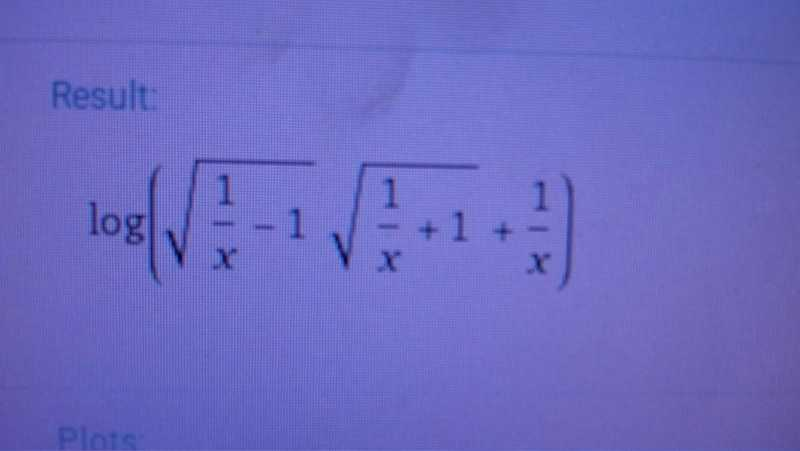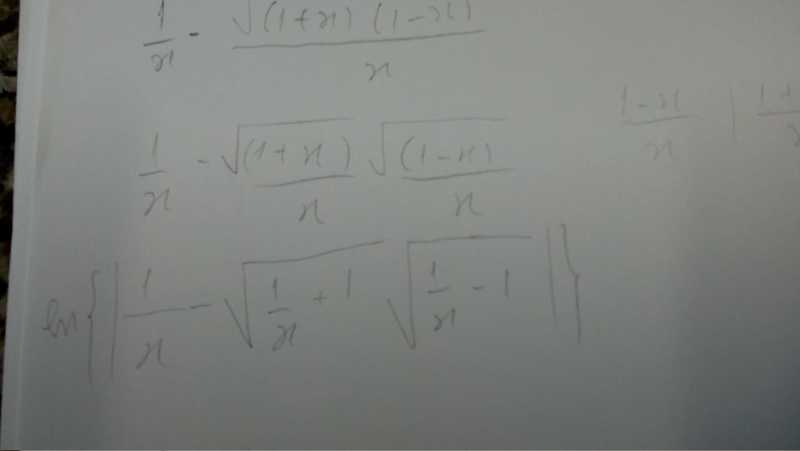# How to integrate this function?

• Mutatis
In summary, the person attempted to solve the equation using two different calculators and both gave the same answer.f

## Homework Statement

I've got to integrate the following $$\int dx =\int \frac {d\phi} {\phi \sqrt {1 - \phi²}}.$$

## Homework Equations

[/B]
I already know the answer but not how to get it. The answer that I got from solution is ## x = \operatorname {arcsech}{\phi} ##.

## The Attempt at a Solution

I've used two apps to help me get this answer. First I did it using Mathway and I got:.

Then, WolframAlpha told me that ## x = \operatorname {arcsech}{\phi} ## is equal to:.

After that I took that first equation in the first image and with some algebraic manipulations I got:.

So I don't know how to procedure beyond here. This was the closest I achieved from the final answer.

## Homework Statement

I've got to integrate the following $$\int dx =\int \frac {d\phi} {\phi \sqrt {1 - \phi²}}.$$

## Homework Equations

[/B]
I already know the answer but not how to get it. The answer that I got from solution is ## x = \operatorname {arcsech}{\phi} ##.

## The Attempt at a Solution

I've used two apps to help me get this answer. First I did it using Mathway and I got:
View attachment 227957 .

Then, WolframAlpha told me that ## x = \operatorname {arcsech}{\phi} ## is equal to:
View attachment 227958 .

After that I took that first equation in the first image and with some algebraic manipulations I got:
View attachment 227959 .

So I don't know how to procedure beyond here. This was the closest I achieved from the final answer.
One approach would be to use a trig substitution, with ##\sin(u) = \sqrt{1 - \phi^2}##, and ##\cos(u)du = \frac{-2\phi d\phi}{\sqrt{1 - \phi^2}}##. The result you get probably won't look like the solution you show, but it should be equal to the solution above within a constant.

I already know the answer but not how to get it. The answer that I got from solution is ## x = \operatorname {arcsech}{\phi} ##.
You dropped a sign somewhere. The derivative of arcsech is the negative of the integrand.

I'd use the substitution ##\phi = \cos u##. It's pretty much the same as Mark's suggestion, but it makes the algebra a little simpler.

Thank you guys! I'm going to try to do it again watching the signs and doing what you've said!